Скачать презентацию Dick Bond Dynamical Trajectories for Inflation now

dfe8d32ed972625e5e1943c7b8f89cd1.ppt

• Количество слайдов: 33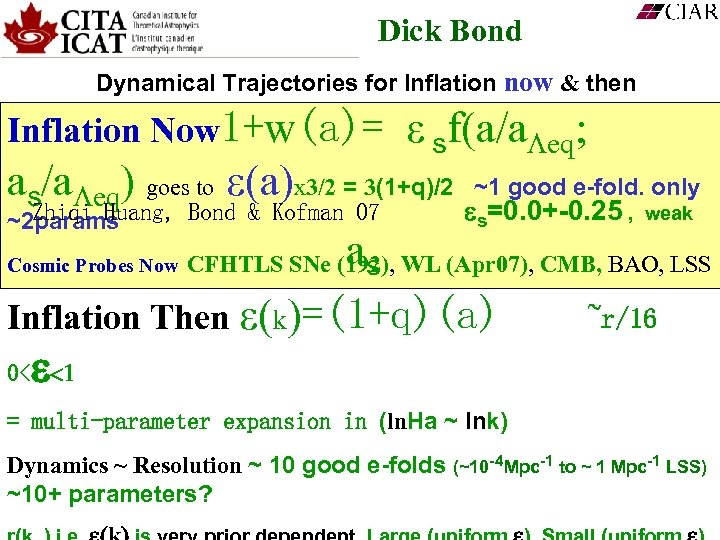Dick Bond Dynamical Trajectories for Inflation now & then Inflation Now 1+w(a)= ε sf(a/a eq; as/a eq) goes to a x 3/2 = 3(1+q)/2 Zhiqi Huang, Bond & Kofman 07 ~2 params ~1 good e-fold. only s=0. 0+-0. 25 , a Inflation Then k =(1+q)(a) 0< Cosmic Probes Now weak CFHTLS SNe (192), WL (Apr 07), CMB, BAO, LSS s ~r/16 = multi-parameter expansion in (ln. Ha ~ lnk) Dynamics ~ Resolution ~ 10 good e-folds (~10 -4 Mpc-1 to ~ 1 Mpc-1 LSS) ~10+ parameters?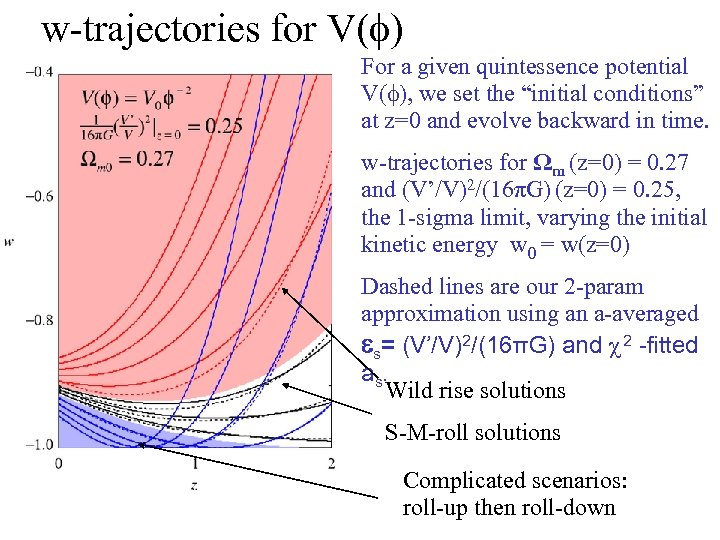w-trajectories for V(f) For a given quintessence potential V(f), we set the “initial conditions” at z=0 and evolve backward in time. w-trajectories for Ωm (z=0) = 0. 27 and (V’/V)2/(16πG) (z=0) = 0. 25, the 1 -sigma limit, varying the initial kinetic energy w 0 = w(z=0) Dashed lines are our 2 -param approximation using an a-averaged s= (V’/V)2/(16πG) and χ 2 -fitted a s. Wild rise solutions S-M-roll solutions Complicated scenarios: roll-up then roll-down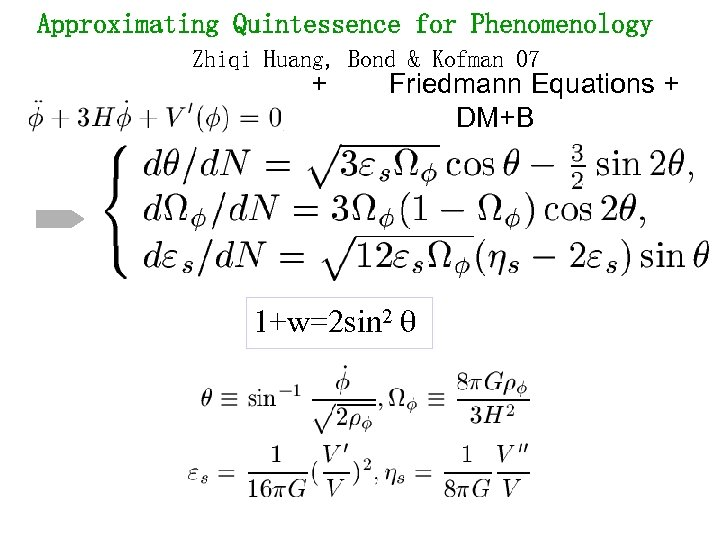Approximating Quintessence for Phenomenology Zhiqi Huang, Bond & Kofman 07 + Friedmann Equations + DM+B 1+w=2 sin 2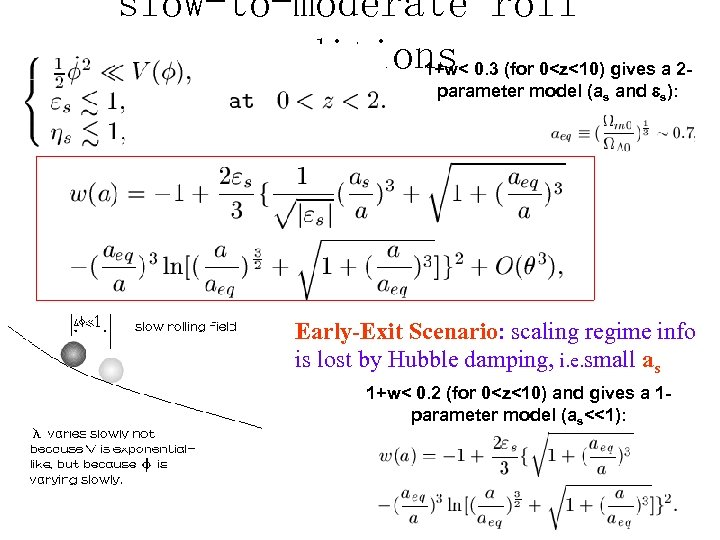slow-to-moderate roll conditions 0. 3 (for 0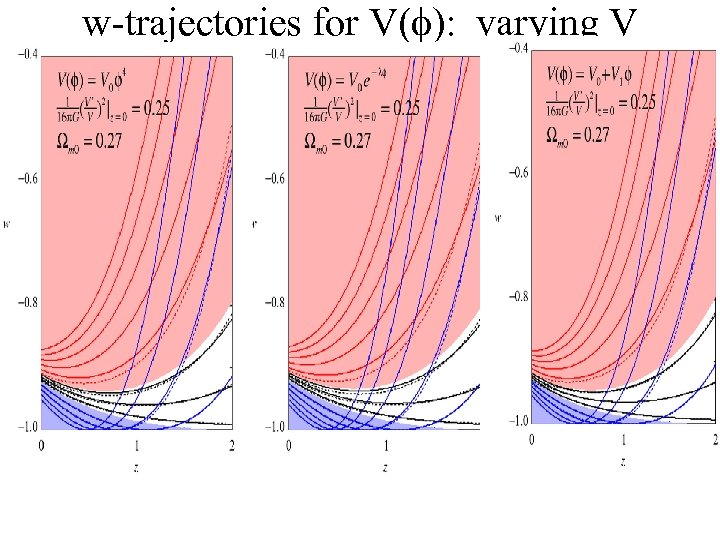w-trajectories for V(f): varying V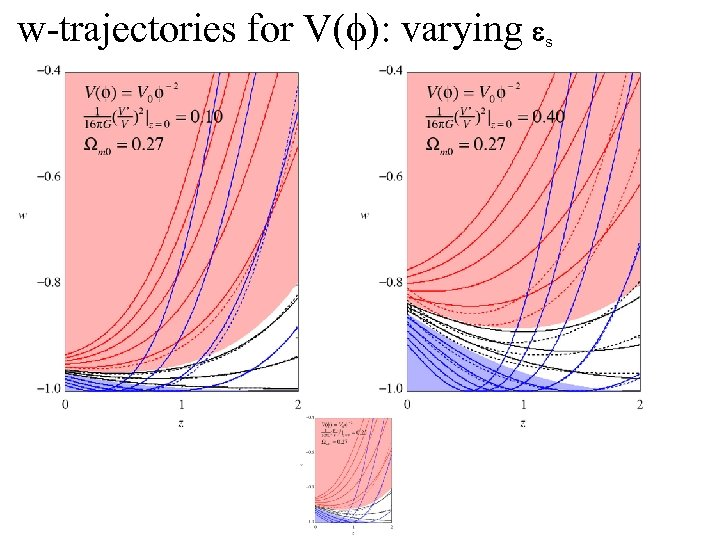w-trajectories for V(f): varying s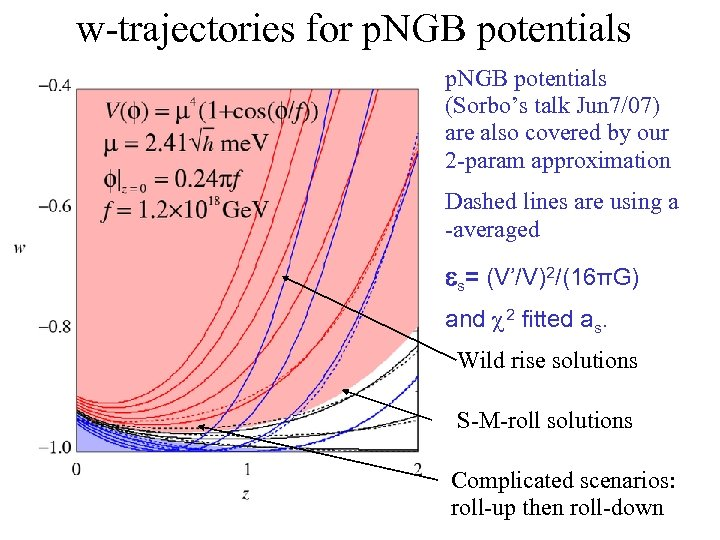w-trajectories for p. NGB potentials (Sorbo’s talk Jun 7/07) are also covered by our 2 -param approximation Dashed lines are using a -averaged s= (V’/V)2/(16πG) and χ 2 fitted as. Wild rise solutions S-M-roll solutions Complicated scenarios: roll-up then roll-down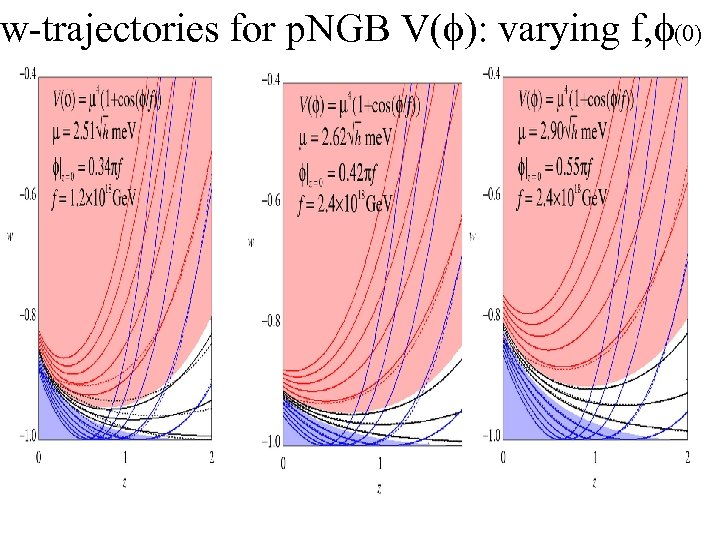w-trajectories for p. NGB V(f): varying f, f(0)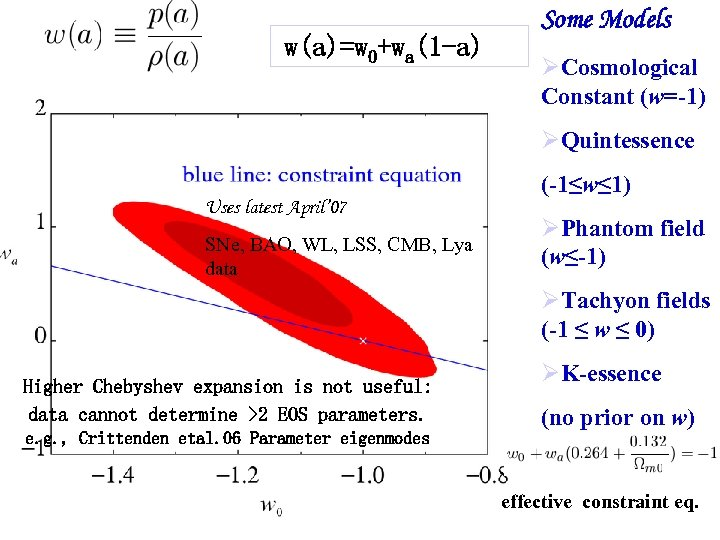w(a)=w 0+wa(1 -a) Some Models Cosmological Constant (w=-1) Quintessence (-1≤w≤ 1) Uses latest April’ 07 SNe, BAO, WL, LSS, CMB, Lya data Phantom field (w≤-1) Tachyon fields (-1 ≤ w ≤ 0) Higher Chebyshev expansion is not useful: data cannot determine >2 EOS parameters. e. g. , Crittenden etal. 06 Parameter eigenmodes K-essence (no prior on w) effective constraint eq.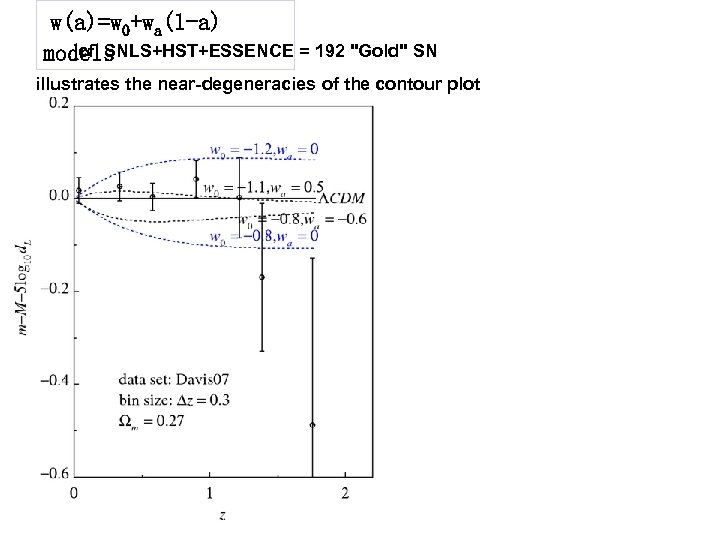w(a)=w 0+wa(1 -a) cf. SNLS+HST+ESSENCE = 192 "Gold" SN models illustrates the near-degeneracies of the contour plot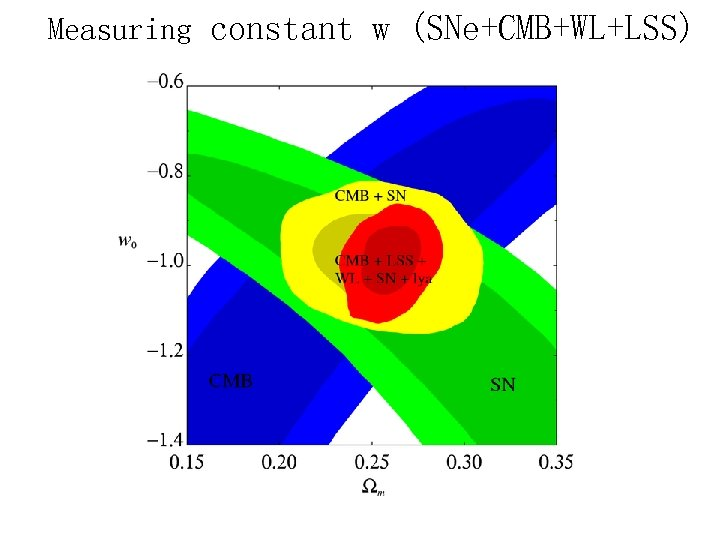Measuring constant w (SNe+CMB+WL+LSS)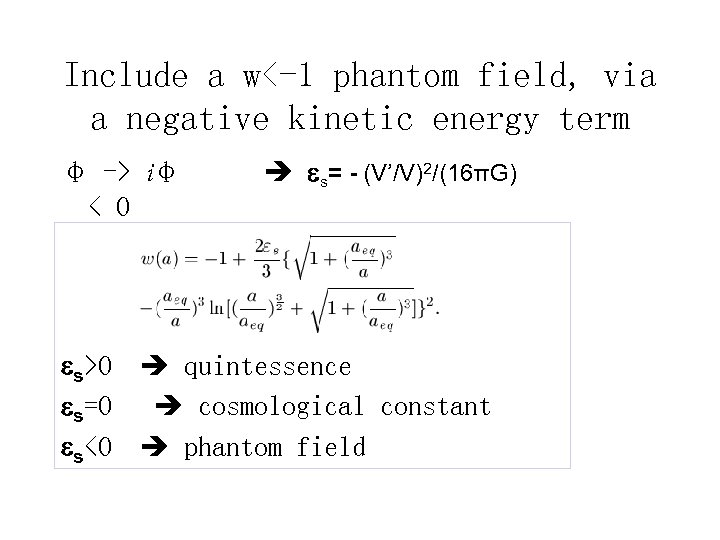Include a w<-1 phantom field, via a negative kinetic energy term φ -> iφ < 0 s= - (V’/V)2/(16πG) s>0 quintessence s=0 cosmological constant s<0 phantom field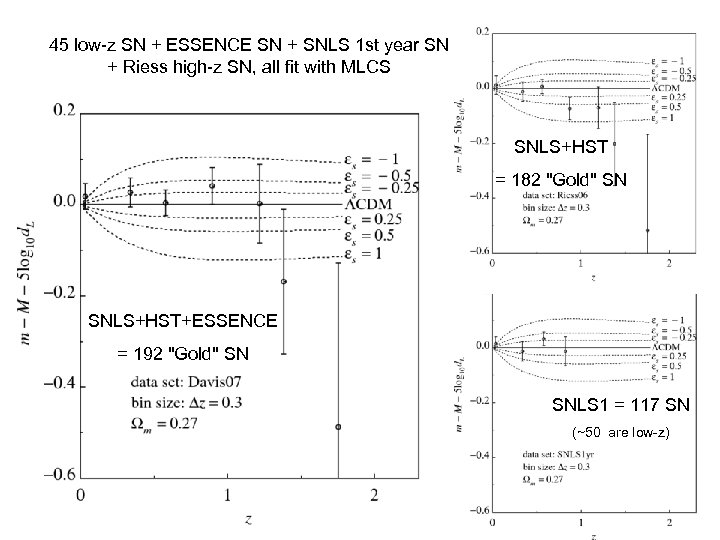45 low-z SN + ESSENCE SN + SNLS 1 st year SN + Riess high-z SN, all fit with MLCS SNLS+HST = 182 "Gold" SN SNLS+HST+ESSENCE = 192 "Gold" SN SNLS 1 = 117 SN (~50 are low-z)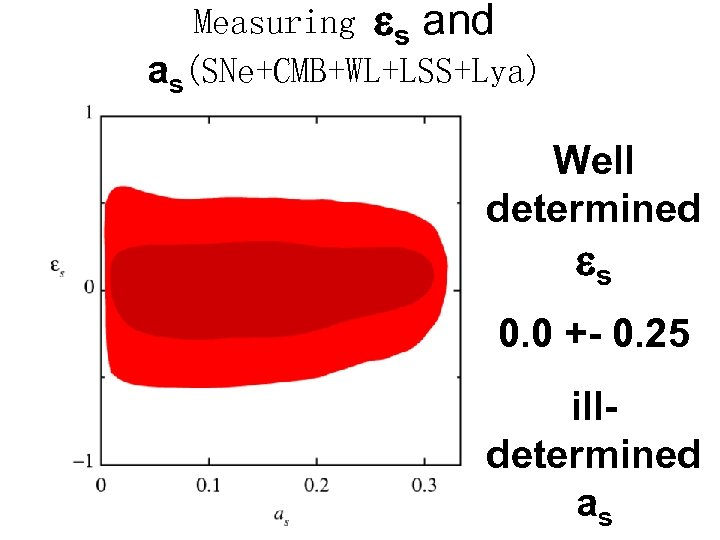Measuring s and as(SNe+CMB+WL+LSS+Lya) Well determined s 0. 0 +- 0. 25 illdetermined as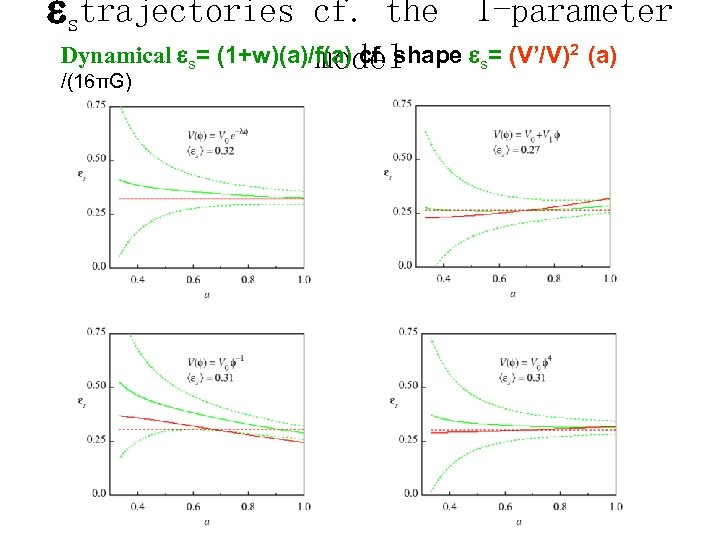strajectories cf. the 1 -parameter Dynamical s= (1+w)(a)/f(a) cf. shape s= (V’/V)2 (a) model /(16πG)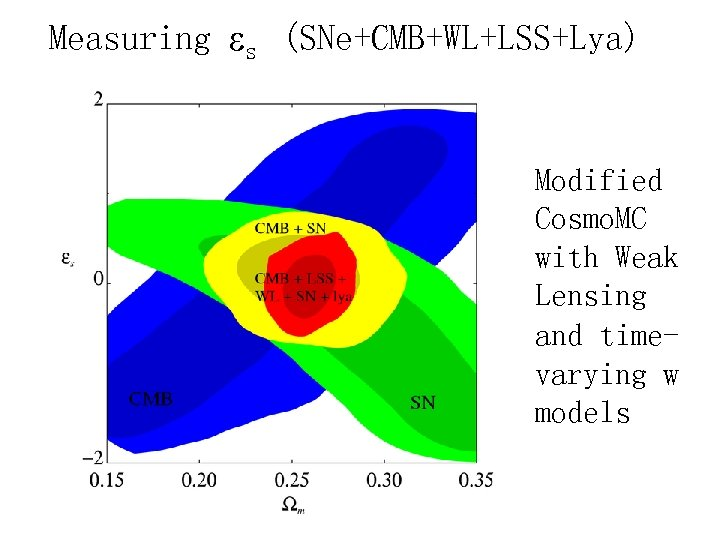Measuring s (SNe+CMB+WL+LSS+Lya) Modified Cosmo. MC with Weak Lensing and timevarying w models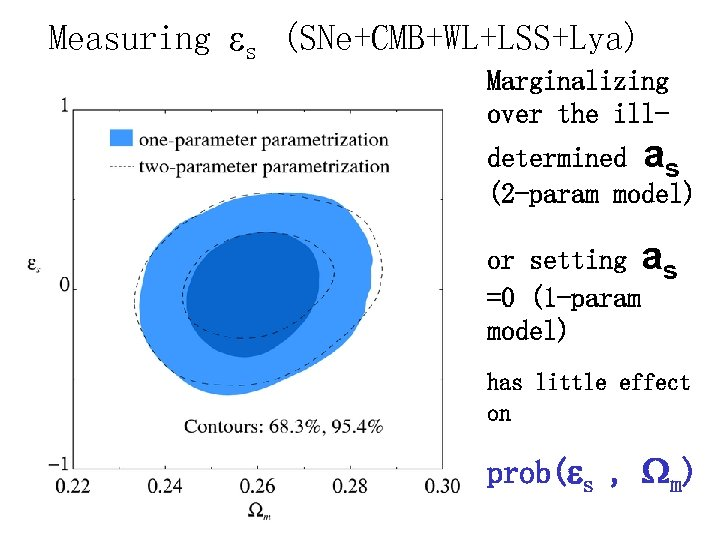Measuring s (SNe+CMB+WL+LSS+Lya) Marginalizing over the illdetermined as (2 -param model) or setting as =0 (1 -param model) has little effect on prob( s , Wm)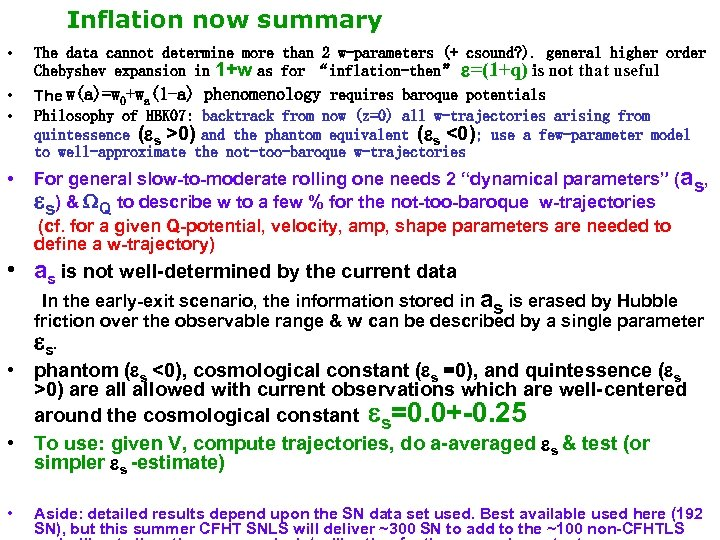Inflation now summary • The data cannot determine more than 2 w-parameters (+ csound? ). general higher order Chebyshev expansion in 1+w as for “inflation-then” =(1+q) is not that useful • • The w(a)=w 0+wa(1 -a) phenomenology requires baroque potentials Philosophy of HBK 07: backtrack from now (z=0) all w-trajectories arising from quintessence ( s >0) and the phantom equivalent ( s <0); use a few-parameter model to well-approximate the not-too-baroque w-trajectories • For general slow-to-moderate rolling one needs 2 “dynamical parameters” ( as, s) & WQ to describe w to a few % for the not-too-baroque w-trajectories (cf. for a given Q-potential, velocity, amp, shape parameters are needed to define a w-trajectory) • as is not well-determined by the current data In the early-exit scenario, the information stored in as is erased by Hubble friction over the observable range & w can be described by a single parameter s. • phantom ( s <0), cosmological constant ( s =0), and quintessence ( s >0) are allowed with current observations which are well-centered around the cosmological constant s=0. 0+-0. 25 • To use: given V, compute trajectories, do a-averaged s & test (or simpler s -estimate) • Aside: detailed results depend upon the SN data set used. Best available used here (192 SN), but this summer CFHT SNLS will deliver ~300 SN to add to the ~100 non-CFHTLS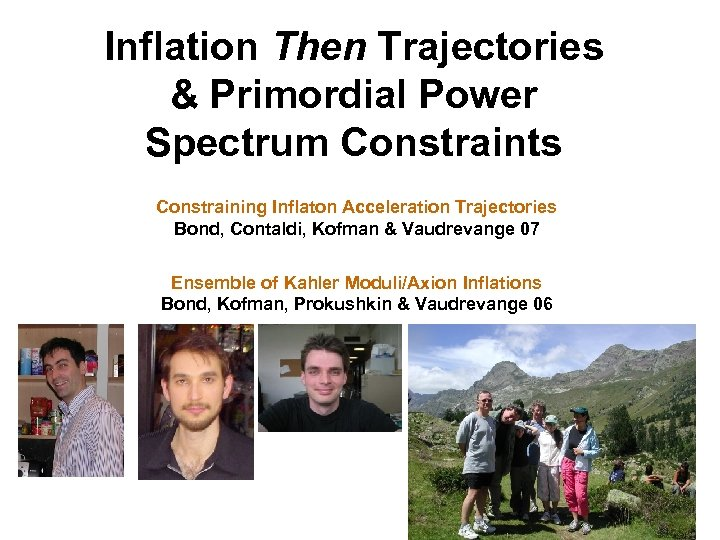Inflation Then Trajectories & Primordial Power Spectrum Constraints Constraining Inflaton Acceleration Trajectories Bond, Contaldi, Kofman & Vaudrevange 07 Ensemble of Kahler Moduli/Axion Inflations Bond, Kofman, Prokushkin & Vaudrevange 06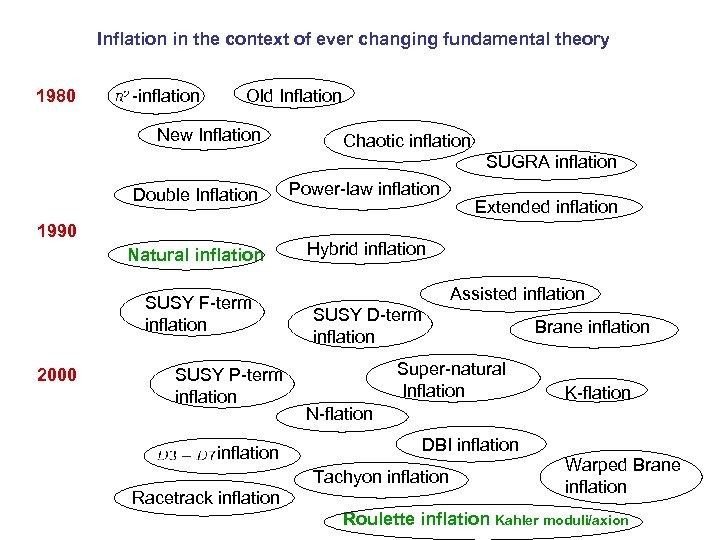Inflation in the context of ever changing fundamental theory 1980 -inflation Old Inflation New Inflation Chaotic inflation SUGRA inflation Double Inflation Power-law inflation Natural inflation Hybrid inflation 1990 SUSY F-term inflation 2000 SUSY P-term inflation Assisted inflation SUSY D-term inflation Super-natural Inflation Brane inflation K-flation N-flation DBI inflation Tachyon inflation Racetrack inflation Extended inflation Warped Brane inflation Roulette inflation Kahler moduli/axion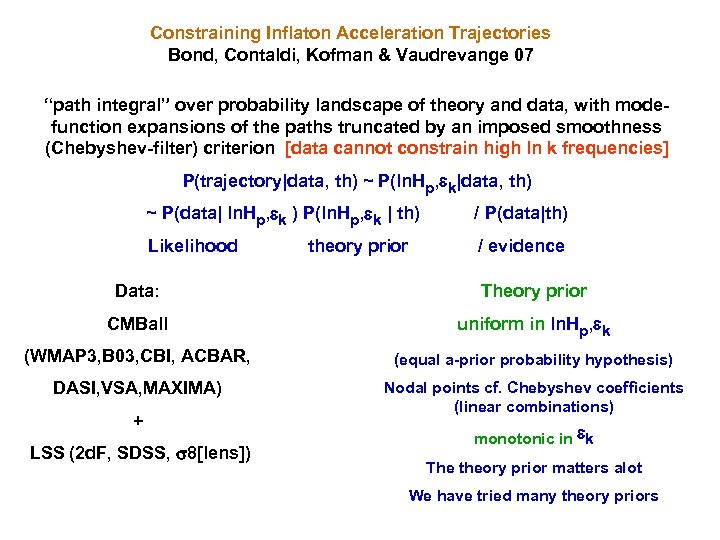Constraining Inflaton Acceleration Trajectories Bond, Contaldi, Kofman & Vaudrevange 07 “path integral” over probability landscape of theory and data, with modefunction expansions of the paths truncated by an imposed smoothness (Chebyshev-filter) criterion [data cannot constrain high ln k frequencies] P(trajectory|data, th) ~ P(ln. Hp, k|data, th) ~ P(data| ln. Hp, k ) P(ln. Hp, k | th) / P(data|th) Likelihood / evidence theory prior Data: Theory prior CMBall uniform in ln. Hp, k (WMAP 3, B 03, CBI, ACBAR, (equal a-prior probability hypothesis) DASI, VSA, MAXIMA) Nodal points cf. Chebyshev coefficients (linear combinations) + LSS (2 d. F, SDSS, 8[lens]) monotonic in k The theory prior matters alot We have tried many theory priors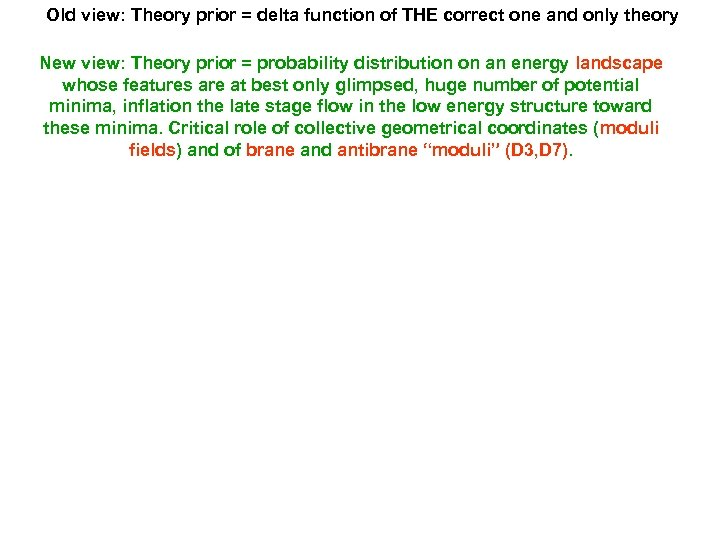Old view: Theory prior = delta function of THE correct one and only theory New view: Theory prior = probability distribution on an energy landscape whose features are at best only glimpsed, huge number of potential minima, inflation the late stage flow in the low energy structure toward these minima. Critical role of collective geometrical coordinates (moduli fields) and of brane and antibrane “moduli” (D 3, D 7).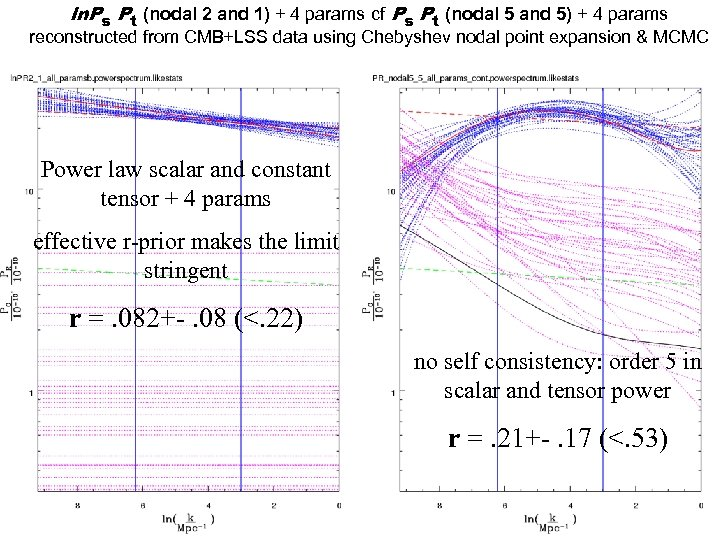ln. Ps Pt (nodal 2 and 1) + 4 params cf Ps Pt (nodal 5 and 5) + 4 params reconstructed from CMB+LSS data using Chebyshev nodal point expansion & MCMC Power law scalar and constant tensor + 4 params effective r-prior makes the limit stringent r =. 082+-. 08 (<. 22) no self consistency: order 5 in scalar and tensor power r =. 21+-. 17 (<. 53)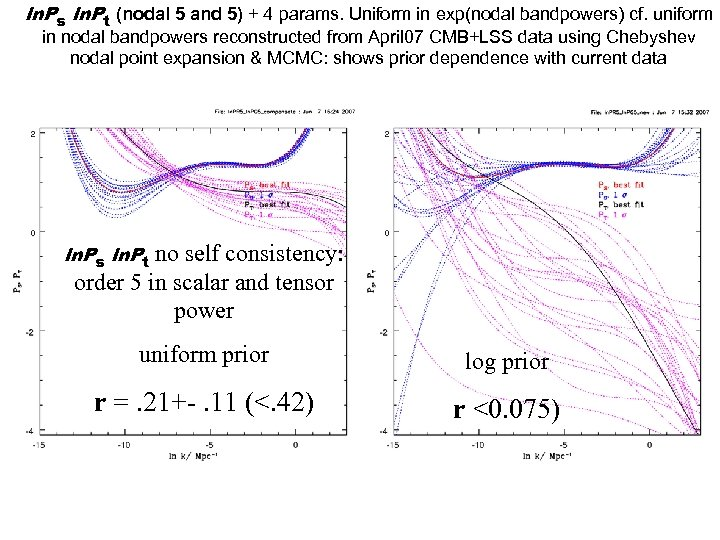ln. Ps ln. Pt (nodal 5 and 5) + 4 params. Uniform in exp(nodal bandpowers) cf. uniform in nodal bandpowers reconstructed from April 07 CMB+LSS data using Chebyshev nodal point expansion & MCMC: shows prior dependence with current data no self consistency: order 5 in scalar and tensor power ln. Ps ln. Pt uniform prior log prior r =. 21+-. 11 (<. 42) r <0. 075)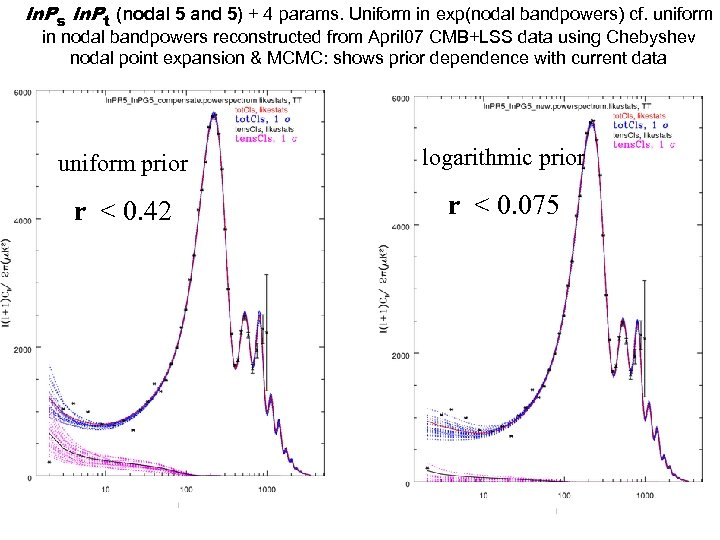ln. Ps ln. Pt (nodal 5 and 5) + 4 params. Uniform in exp(nodal bandpowers) cf. uniform in nodal bandpowers reconstructed from April 07 CMB+LSS data using Chebyshev nodal point expansion & MCMC: shows prior dependence with current data uniform prior logarithmic prior r < 0. 42 r < 0. 075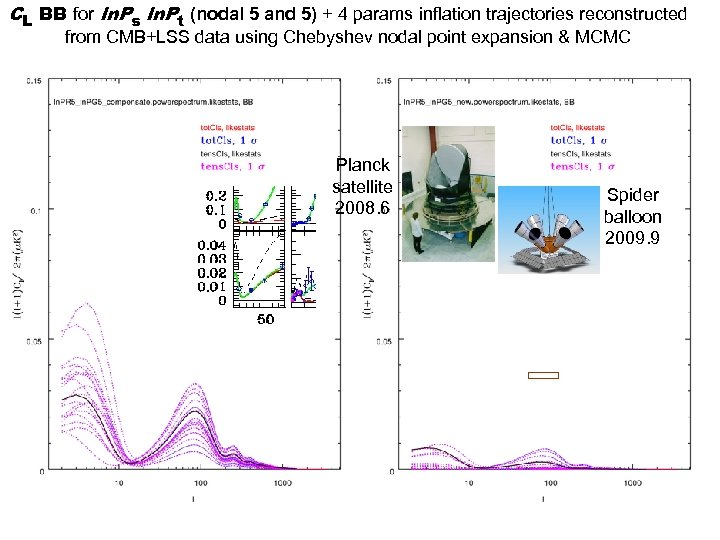CL BB for ln. Ps ln. Pt (nodal 5 and 5) + 4 params inflation trajectories reconstructed from CMB+LSS data using Chebyshev nodal point expansion & MCMC Planck satellite 2008. 6 Spider balloon 2009. 9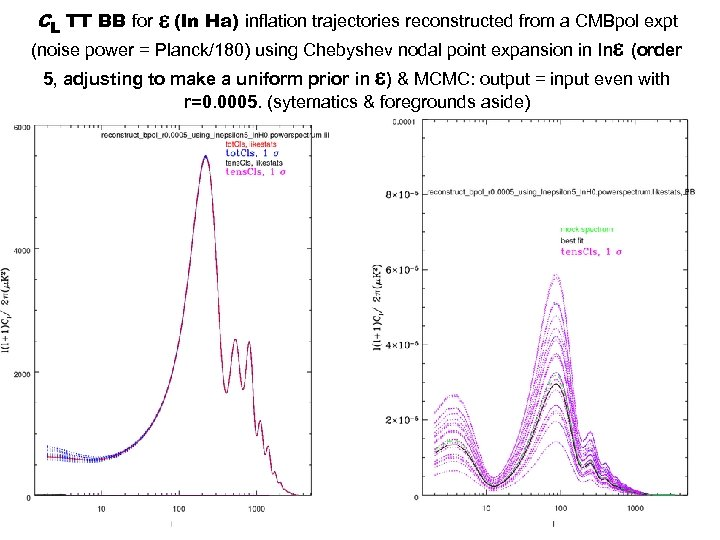(ln Ha) inflation trajectories reconstructed from a CMBpol expt (noise power = Planck/180) using Chebyshev nodal point expansion in ln (order 5, adjusting to make a uniform prior in ) & MCMC: output = input even with CL TT BB for r=0. 0005. (sytematics & foregrounds aside)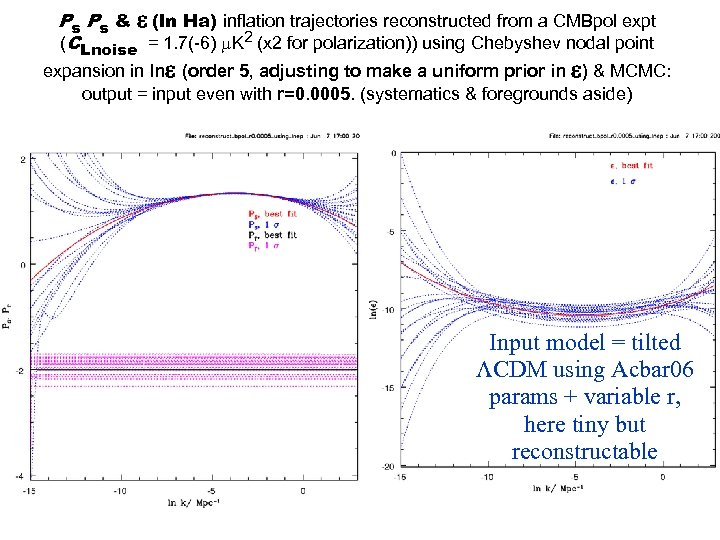Ps Ps & (ln Ha) inflation trajectories reconstructed from a CMBpol expt (CLnoise = 1. 7(-6) m. K 2 (x 2 for polarization)) using Chebyshev nodal point expansion in ln (order 5, adjusting to make a uniform prior in ) & MCMC: output = input even with r=0. 0005. (systematics & foregrounds aside) Input model = tilted CDM using Acbar 06 params + variable r, here tiny but reconstructable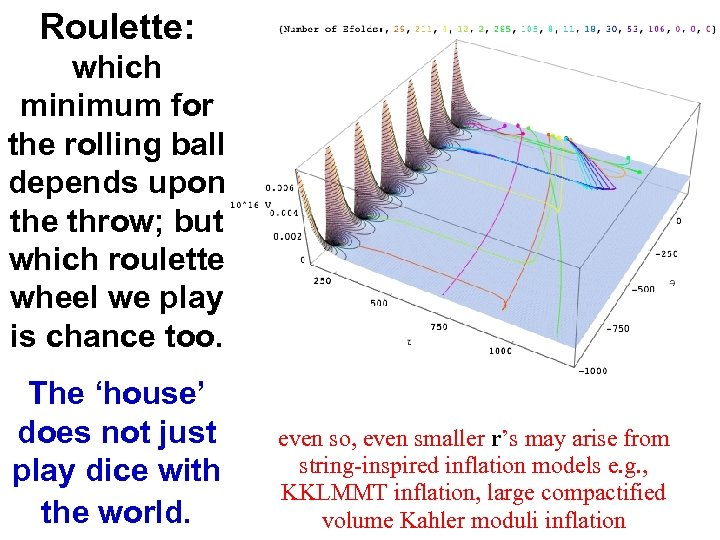Roulette: which minimum for the rolling ball depends upon the throw; but which roulette wheel we play is chance too. The ‘house’ does not just play dice with the world. even so, even smaller r’s may arise from string-inspired inflation models e. g. , KKLMMT inflation, large compactified volume Kahler moduli inflation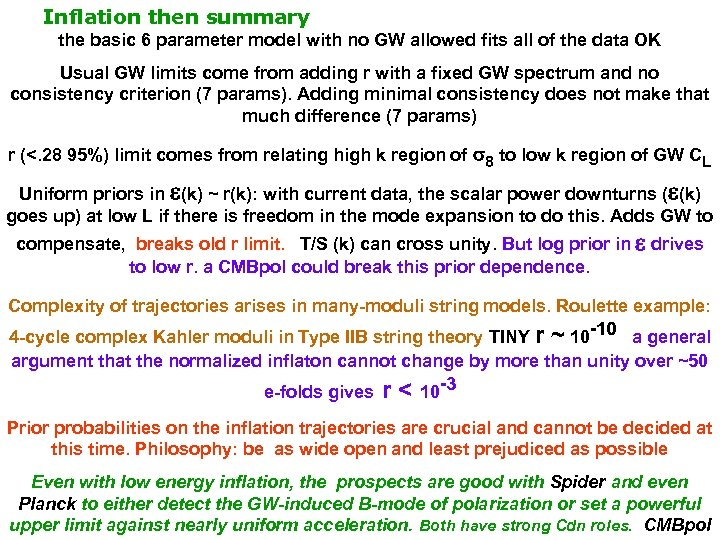Inflation then summary the basic 6 parameter model with no GW allowed fits all of the data OK Usual GW limits come from adding r with a fixed GW spectrum and no consistency criterion (7 params). Adding minimal consistency does not make that much difference (7 params) r (<. 28 95%) limit comes from relating high k region of 8 to low k region of GW CL Uniform priors in (k) ~ r(k): with current data, the scalar power downturns ( (k) goes up) at low L if there is freedom in the mode expansion to do this. Adds GW to compensate, breaks old r limit. T/S (k) can cross unity. But log prior in drives to low r. a CMBpol could break this prior dependence. Complexity of trajectories arises in many-moduli string models. Roulette example: 4 -cycle complex Kahler moduli in Type IIB string theory TINY r ~ 10 -10 a general argument that the normalized inflaton cannot change by more than unity over ~50 e-folds gives r < 10 -3 Prior probabilities on the inflation trajectories are crucial and cannot be decided at this time. Philosophy: be as wide open and least prejudiced as possible Even with low energy inflation, the prospects are good with Spider and even Planck to either detect the GW-induced B-mode of polarization or set a powerful upper limit against nearly uniform acceleration. Both have strong Cdn roles. CMBpolend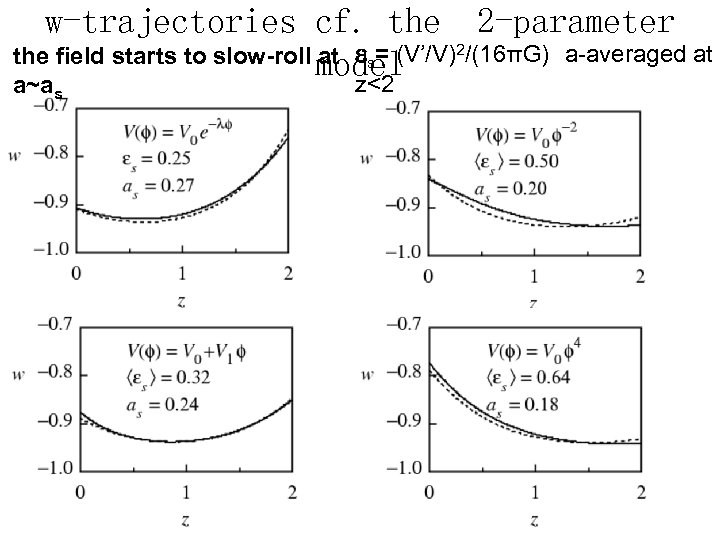w-trajectories cf. the 2 -parameter the field starts to slow-roll model at s= (V’/V)2/(16πG) a-averaged at z<2 a~a s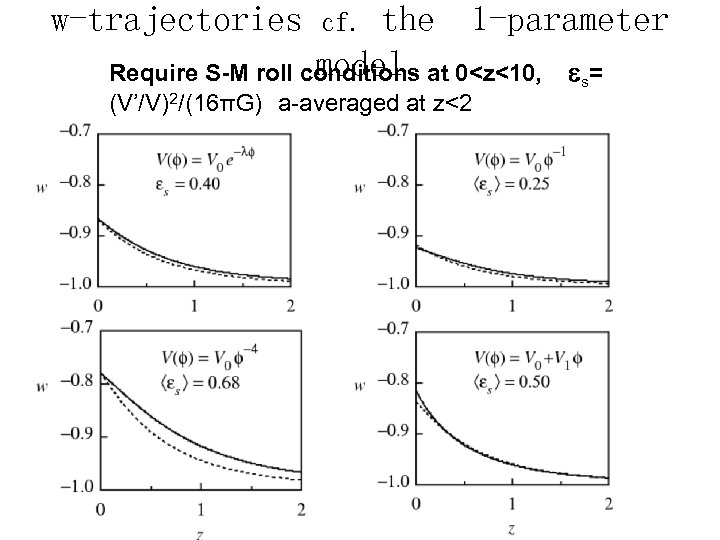w-trajectories the 1 -parameter model Require S-M roll conditions at 0